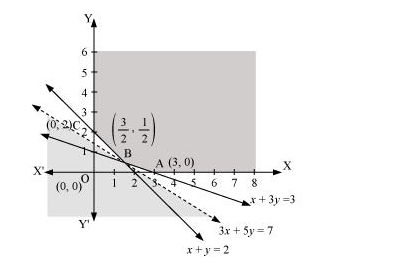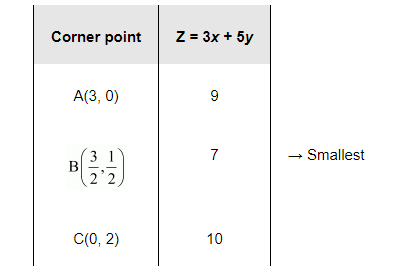# Minimise

Question:

Minimise $Z=3 x+5 y$

such that $x+3 y \geq 3, x+y \geq 2, x, y \geq 0$.

Solution:

The feasible region determined by the system of constraints, $x+3 y \geq 3, x+y \geq 2$, and $x, y \geq 0$, is as follows.It can be seen that the feasible region is unbounded.

The corner points of the feasible region are $A(3,0), B\left(\frac{3}{2}, \frac{1}{2}\right)$, and $C(0,2)$.

The values of Z at these corner points are as follows.As the feasible region is unbounded, therefore, 7 may or may not be the minimum value of Z.

For this, we draw the graph of the inequality, $3 x+5 y<7$, and check whether the resulting half plane has points in common with the feasible region or not.

It can be seen that the feasible region has no common point with $3 x+5 y<7$ Therefore, the minimum value of $Z$ is 7 at $\left(\frac{3}{2}, \frac{1}{2}\right)$.